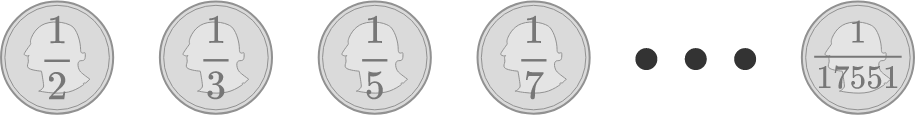# Bijections Galore

Probability Level 1

Ram has 2018 coins.

The $1^\text{st}$ coin has a $\frac{1}{2}$ chance of flipping heads, the $2^\text{nd}$ coin has a $\frac{1}{3}$ chance of flipping heads, the $3^\text{rd}$ coin has a $\frac{1}{5}$ chance of flipping heads, continuing such that the $k^\text{th}$ coin has a $\frac{1}{p(k)}$ chance of flipping heads, where $p(k)$ is the $k^\text{th}$ prime number.

Ram flips each of his coins once.

What is the probability that Ram flips an even number of heads?×